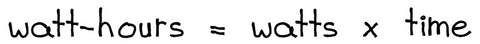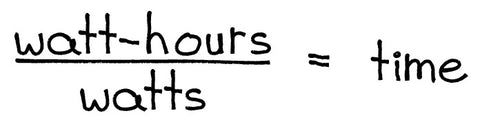-
| /
Save up to %
Save %
Save up to
Save
Sale
Sold out
In stock
0

August 11, 2022 4 min read

How much power can this battery store?

How long will it take to charge?

How long can it run my appliances?

If you’ve ever been in the market for a portable power station, you’ve probably asked these questions. After all, some of the most important factors for determining whether a power station is the right fit for you are storage capacity, charge time, and run-time.

But as you seek these answers, you’re likely to encounter an unfamiliar term: watt-hour. It is a fundamental unit of measure in electricity, but one you’re unlikely to have come across.

In this watt-hour guide, we’ll give you a crash course on what they are and how to use them. Trigger warning: there’s a little math, but I promise it’s straightforward; and if you can sit through it, you’ll be fully equipped to understand battery performance.

## A what hour?

Watt-hour. To understand watt-hours, you first need to know what a watt is.

Watts are a measurement used to quantify electricity; the same way gallons quantify water. It's a standard measurement, so power consumption is always referred to in watts.

Unlike gallons, however, it’s hard to visualize how much electricity is in a watt, so we always talk about watts in relation to time. For example, your fridge might consume 250 wattsper hour, or a solar panel might be rated to produce 100 wattsper hour.

Because power usage is measured by the hour, we need a corresponding measurement for power storage.

Enter the watt-hour.

## So, what is a watt-hour?

Watt-hours (abbreviated as Wh) are used to measure power storage. They’re derived from this equation:Basically, how many watts are used or produced in a length of time.

To standardize this and make the measurement easier to use,watt-hours always refer to how much power is used/produced in one hour.

So that shiny new 3200Wh battery you’re looking at buying would theoretically let you draw power at a rate of 3200W for one hour before it needs a recharge.

But what if you don’t want to use 3200W of power? What if you want to use more, or less? This is where we get to the real meat (and the math).

Watt-hours are a ratio represented by the equation above, and we can use that equation to determine run-times and charge times forany amount of power. I’ll walk you through it.

## How to use watt-hours

If you already know what your power needs are, you can use the watt-hour equation to calculate what size battery you’ll need. For example, if you need to be able to run your home office for 8 hours, you can multiply the total watts used by your home office (just add up the individual wattages for each appliance) by 8 hours. The result watt-hours will tell you how big your battery needs to be.

We can also rewrite the watt-hour equation to tell us how long a battery will last depending on how much power you’re using. It looks like this:So, watt-hours divided by watts equals time. Isn’t math fun?

To use this equation, just plug in the listed Wh of whatever battery you’re looking at and the total wattage of the devices you’re using. The result will be the expected runtime for those devices with that battery.

The same applies for charge time. Divide a battery’s watt-hours by the total wattage of your solar panel array, and the result is how long it will take to charge with those panels.

Finally, we can flip this equation to tell us the maximum number of watts we can use to keep the battery running for a certain length of time, which may be useful for rationing power during a long outage.Divide the battery’s watt-hour rating by the number of hours you’d like the battery to last, and the result is the maximum amount of power you can draw without draining the battery too quickly.

## Conclusion

It’s said that knowledge is power. Here at Inergy, we’re all about empowering you. Knowing how to use watt-hours is incredibly useful, not just for buying a battery, but to help you get the most out of it.

For one, it will help you buy the right battery based on your needs, not just a sales recommendation. You’ll also be able to model different power scenarios, so you know what to expect from your battery before you need it. Finally, you can use this knowledge to make adjustments on the fly, so you can maximize your power output even in an unknown situation.

I know math can be annoying and easy to forget. To help you remember, we’ve put together this watt-hour cheat-sheet. Download it and keep it on your phone or print it out and tape to your battery if you want. Whatever you do, you won't regret knowing how watt-hours work.

﻿Written by: Eitan Mizrahi